# KSEEB Solutions for Class 9 Maths Chapter 12 Circles Ex 12.6

In this chapter, we provide KSEEB SSLC Class 9 Maths Chapter 12 Circles Ex 12.6 for English medium students, Which will very helpful for every student in their exams. Students can download the latest KSEEB SSLC Class 9 Maths Chapter 12 Circles Ex 12.6 pdf, free KSEEB SSLC Class 9 Maths Chapter 12 Circles Ex 12.6 pdf download. Now you will get step by step solution to each question.

## Karnataka Board Class 9 Maths Chapter 12 Circles Ex 12.6

Question 1.
Prove that the line of centres of two intersecting circles subtends equal angles at the two points of intersection.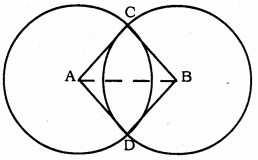Solution:
Data: Two circles having centres A and B, intersect at C and D.
Construction: Join A and B.
Proof: In ∆ABC and ∆ABD,
BC = DD
AB is common.
∴ ∆ABC ≅ ∆ABD (SSS Postulate.)

Question 2.
Two chords AB and CD of lengths 5 cm and 11 cm respectively of a circle are parallel to each other and are on opposite sides of its centre. If the distance between AB and CD is 6 cm., find the radius of the circle.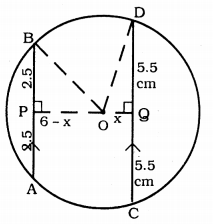Solution:
Two chords AB and CD of lengths 5 cm and 11 cm respectively of a circle are parallel to each other and are on opposite sides of its centre.
Distance between AB and CD is 6 cm.
To Prove: Radius of the circle, OP =?
Construction: Join OP and OQ, OB, and OD.
Proof: Chord AB || Chord CD.
AB = 5 cm, and CD =11 cm.
OP ⊥ AB
∴ BP = AP = 52 = 2.5 cm.
OQ⊥CD
∴ CQ = QD = 112 = 5.5 cm.
PQ = 6 cm. (Data)
Let OQ = 2 cm then, OP = (6 – x) cm.
In ∆BPO, ∠P = 90°
As per Pythagoras theorem,
OB2 = BP2 + PO2
= (2.5)2 + (6 – x)2
= 6.25 + 36 – 12x + x2
OB2 = x2– 12x + 42.25 …………….. (i)
In ∆OQD, ∠Q= 90°
∴ OD2 = OQ2 + QD2
= (x)2 + (5.5)2
OD2 = x2 + 30.25 ……………….. (ii)
OB = OD (∵ radii of same circle)
From (i) and (ii).
x2 – 12x + 42.25 = x2 + 30.25
-12x = 30.25 – 42.25
-12x = -12
12x = 12
∴ x = 1212
∴ x = 1 cm.
From (ii),
OD2 = x2 + 30.25
= (1)2 + 30.25
= 1 + 30.25
∴ OD2 = 31.25
OD = 31.25−−−−√
∴ OD = 5.59 cm.
∴ Radius of circle OP = OD = 5.59 cm.

Question 3.
The lengths of two parallel chords of a circle are 6 cm and 8 cm. If the smaller chord is at distance 4 cm, from the centre, what is the distance of the other chord from the center?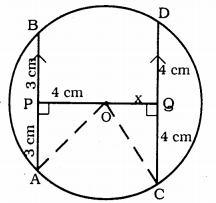Solution:
Data: Chords of a circle are 6 cm. and 8 cm. are parallel. The smaller chord is at distance of 4 cm. from the centre.
To Prove: Distance between bigger chord and centre =?
Construction: Join OA and OC.
Proof: AB || CD, AB = 6 cm, CD = 8 cm.
OP⊥CD, OQ⊥CD.
In ∆OPA, ∠P = 90°
∴ OA2 = OP2 + PA2 (According to Pythagoras theorem)
= (4)+ (3)2 = 16 + 9
OA2 = 25
∴ OA = 5 cm.
OA = OC = 5 cm. (radii of the same circle.)
Now, in ∆OQC,
OC2 = OQ2 + QC2
(5)2 = x2 + (4)2
25 = x2 + 16
x2 = 25 – 16 = 9
∴ x = 9–√ ∴ x = 3 cm.
∴ Bigger chord is at a distance of 3 cm from the centre.

Question 4.
Let the vertex of an angle ABC be located outside a circle and let the sides of the angle intersect equal chords AD and CE with the circle. Prove ∠ABC is equal to half the difference of the angles subtended by the chords AC and DE at the centre.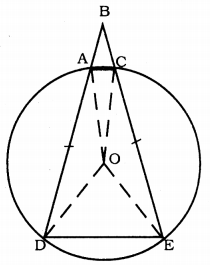Solution:
Data: The vertex of angle ABC be located outside a circle and let the sides of the angle intersect equal chords AD and CE with the circle.
To Prove: ∠ABC is equal to half the difference of the angles subtended by the chords AC and DE at the centre. OR
∠ABC= 12 [∠DOE – ∠AOC].
Construction: OA, OC, OE, OD are joined.
Proof: In ∆AOD and ∆COE,
OA = OC, OD = OE radii of same circle.
∴ ∆AOD ≅ ∆COE (SSS Postulate)
∴∠ODA = ∠OEC …………. (ii)
OA = OD
∴ ∠OAD = ∠ODA …………. (iii)
From (i) and (ii),
∠OAD = ∠OCE = ∠ODA = ∠OEC = x°.
In ∆ODE, OD = OE
∠ODE = ∠OED = y°.
∴ ∠CAD + ∠DEC = 180°
x + a + x + y = 180
2x + a + y = 180
y = 180 – 2x – a ……….. (iv)
But, ∠DOE = 180 – 2y
∠AOC = 180 – 2a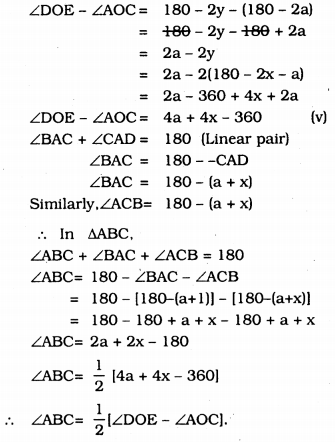Question 5.
Prove that the circle drawn with any side of a rhombus as diameter passes through the point of intersection of its diagonals.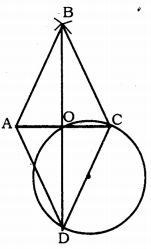Solution:
Data: ABCD is a rhombus. Circle is drawn taking side CD diameter. Let the diagonals AC and BD intersect at ‘O’.
To Prove: Circle passes through the point ‘O’ of the intersection of its diagonals.
Proof: ∠DOC = 90° (Angle in the semicircle) and diagonals of rhombus bisect at right angles at ‘O’.
∴ ∠DOC = ∠COB = ∠BOA = ∠AOD = 90°
∴ Circle passes the point of intersection of its diagonal through ‘O’.

Question 6.
ABCD is a parallelogram. The circle through A, B and C intersect CD (produced if necessary) at E. Prove that AE = AD.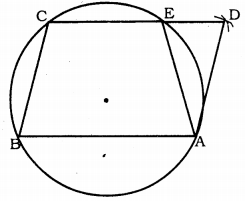Solution:
Data: ABCD is a parallelogram. The circle through A, B, and C intersect CD at E. AE is joined.
Proof: ∠AEC + ∠AED = 180° …………. (i) (linear pair)
∴ ∠ABC + ∠AEC = 180° ………….(ii) (opposite angles)
Comparing (i) and (ii),
∠AEC = ∠AED = ∠ABC + ∠AEC
∠AED = ∠ABC ………….. (iii)
Substituting in equation (iii),

Question 7.
AC and BD are chords of a circle which bisect each other. Prove that
(i) AC and BD are diameters,
(ii) ABCD is a rectangle.
Solution:
Data : AC and BD are chords of a circle bisect each other at ‘O’.
To Prove:
i) AC and BD are diameters.
ii) ABCD is a rectangle.
Construction: AB, BC, CD and DA are joined.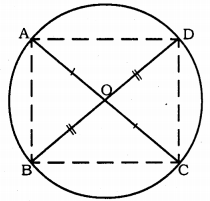Proof: In ∆AOB and ∆COD,
AO = OC (Data)
BO = OD (Data)
∠AOB = ∠COD (vertically opposite angles)
∴ ∆AOB ≅ ∆COD (SAS postulate)
∴ ∠OAB = ∠OCD
These are pair of alternate angles.
∴ AB || CD and AB = CD.
∴ ABCD is a parallelogram.
∴ ∠BAD = ∠BCD (Opposite angles of parallelogram)
If angles of a quadrilateral are right angles it is rectangle. ABCD is a recrtangle.
∠BAD is separated from chord BD.
∴ This is the angl in semicircle.
∴ Chord BD is a diameter.
∴ Chord AC is a diameter.

Question 8.
Bisectors of angles A, B and C of a triangle ABC intersect its circumcircle at D, E and F respectively. Prove that the angles of the triangle DEF are 90° – 12 A, 90° – 12 B and 90° – 12 C.
Solution:
Data: AD, BE and CF are angular bisectors of angles A, B and C of ∆ABC intersects its circumference at D, E and F respectively.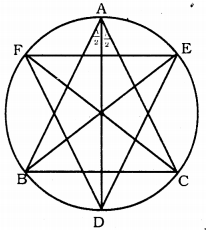To prove: Angles of ∆DEF are 90° – 12 A, 90° – 12 B and 90° – 12 C.
Proof: AD, BE and CF are angular bisectors of angles A, B and C of ∆ABC.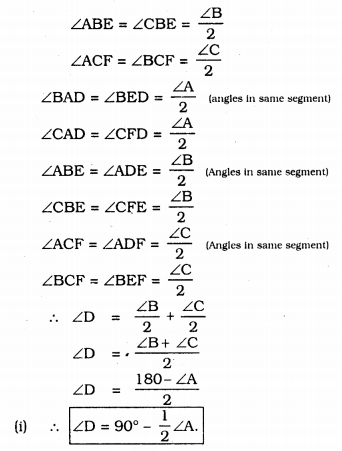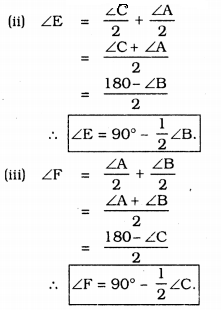Question 9.
Two congruent circles intersect each other at points A and B. Through A any line segment PAQ is drawn so that P, Q lies on the two circles.
Prove that BP = BQ.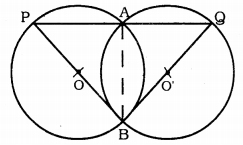Solution:
Data : Two congruent circles intersect each other at points A and B. Through A any line segment PAQ is drawn so that P, Q lie on the two circles.
To Prove: BP = BQ
Construction: Join AB.
Proof: Two congruent triangles with centres O and O’ intersects at A and B. Through A segment PAQ is drawn so that P, Q lie on the two circles.
Similarly, ∠AQB= 70° in circle subtended by chord AB. Because Angles subtended by circumference by same chord.
∴ ∠APB = ∠AQB = 70°.
Now, in ∆PBQ, ∠QPB = ∠PQB.
∴ Sides opposite to each other are equal.
∴ BP = BQ.

Question 10.
In any triangle ABC, if the angle bisector of ∠A and perpendicular bisector of BC intersect, prove that they intersect on the circumcircle of the triangle ABC.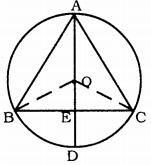Solution:
Data: In ∆ABC, if the angle bisector of ∠A and perpendicular bisector of BC intersect each other. O is the centre of the circle.
To Prove: Angle bisector of ∠A and perpendicular bisector of BC intersect at D.
Construction: Join OB, OC.
Proof: Angle subtended at the Centre
= 2 × angles subtended in the circumference.
∠BOC = 2 × ∠BAC
In ∆BOE and ∆COE,
∠OEB = ∠OEC = 90° (∵ OE⊥BC)
OE is common.
∴ ∆BOE ≅ ∆COE (RHS postulate)
But, ∠BOE + ∠COE = ∠BOC
∠BOE + ∠BOE = ∠BOC
2∠BOE = ∠BOC
2∠BOE = 2∠BAC
∴ ∠BOE = ∠BAC
But, ∠BOE = ∠COE = ∠BAC
∴ The angle subtended by an arc at the centre is double the angle subtended by it at any point on the circumference.
∴ Angle bisector of ∠A and perpendicular bisector of BC intersect at D.

All Chapter KSEEB Solutions For Class 9 Maths

—————————————————————————–

All Subject KSEEB Solutions For Class 9

*************************************************

I think you got complete solutions for this chapter. If You have any queries regarding this chapter, please comment on the below section our subject teacher will answer you. We tried our best to give complete solutions so you got good marks in your exam.

If these solutions have helped you, you can also share kseebsolutionsfor.com to your friends.

Best of Luck!!